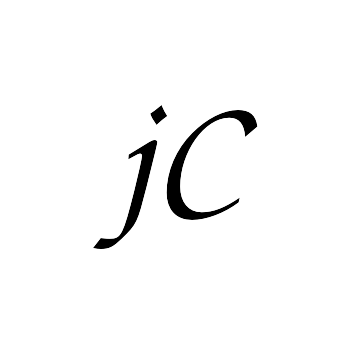# jsCanvas Help

## Tutorials

### Maths

These tutorials are aimed at exploring specific topics in mathematics.

• Variables

This tutorial shows how to set variables and use them with algebra to do calculations.

• Coordinates

This tutorial introduces tables and iteration by making a coordinate drawing program.

• Lines

This tutorial introduces conditionals by testing whether a point lies on a line.

• Angles

This tutorial introduces angles using the `Logo` programming language.

### Programming

These tutorials introduce various programming concepts and aspects of the jsCanvas system.

## Examples

• Grid

This is the template for the tutorial on Coordinates. It draws a grid on the screen and sets up the transformation to match the grid.

• Coordinates

This is the end result of the Coordinates tutorial.

• MakeCoordinates

This example is a counterpart to the Coordinates example. It draws a grid on which the user can create coordinate pictures by clicking.

• Spirolaterals

This example draws spirolaterals which are diagrams created by mixing times tables and modular arithmetic.

• Cellular

This example is an implementation of cellular automata.

• CurveStitch

This example draws curve stitching diagrams where the points lie on a circle and each point is joined to a fixed multiple of it.

• Game of Life

This shows Conway's Game of Life in a finite sized grid.

• Worms

This shows the Worms simulation.